CS2113/T AY1819S1
• Nested (current format)
•     Flat
• Schedule
• Textbook
• Report Bugs
• Slack
• Forum
• Instructors
• IVLE Announcements
• IVLE File Submissions
• Tutorial Schedule
• Java Coding Standard
• samplerepo-things
• Projects List
• Code Dashboard (BETA)
• # Modeling

## Introduction

Can explain models

A model is a representation of something else.

A class diagram is a model that represents a software design.

A class diagram is a diagram drawn using the UML modelling notation.
An example class diagram: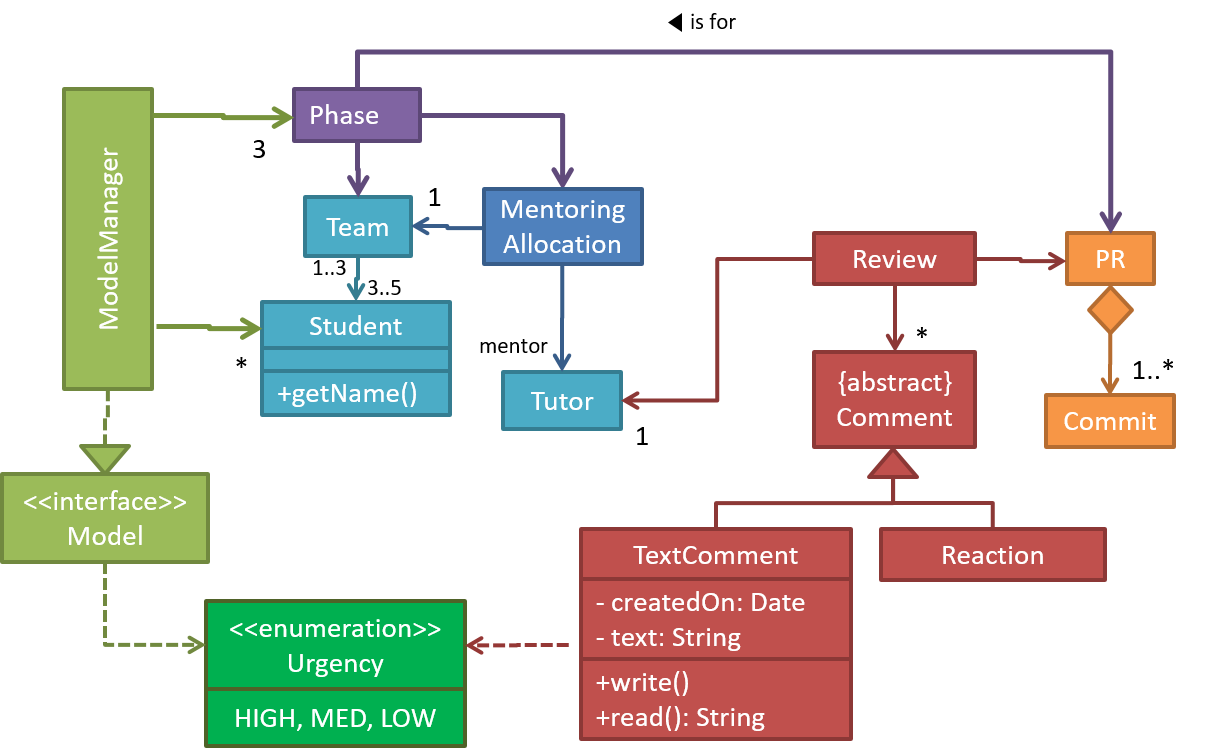A model provides a simpler view of a complex entity because a model captures only a selected aspect. This omission of some aspects implies models are abstractions.

Design → Design Fundamentals → Abstraction →

#### What

Abstraction is a technique for dealing with complexity. It works by establishing a level of complexity (or an aspect) we are interested in, and suppressing the more complex details below that level (or irrelevant to that aspect).

Most programs are written to solve complex problems involving large amounts of intricate details. It is impossible to deal with all these details at the same time. The guiding principle of abstraction stipulates that we capture only details that are relevant to the current perspective or the task at hand.

Ignoring lower level data items and thinking in terms of bigger entities is called data abstraction.

Within a certain software component, we might deal with a user data type, while ignoring the details contained in the user data item such as name, and date of birth. These details have been ‘abstracted away’ as they do not affect the task of that software component.

Control abstraction abstracts away details of the actual control flow to focus on tasks at a simplified level.

`print(“Hello”)` is an abstraction of the actual output mechanism within the computer.

Abstraction can be applied repeatedly to obtain progressively higher levels of abstractions.

An example of different levels of data abstraction: a `File` is a data item that is at a higher level than an array and an array is at a higher level than a bit.

An example of different levels of control abstraction: `execute(Game)` is at a higher level than `print(Char)` which is at a higher than an Assembly language instruction `MOV`.

A class diagram captures the structure of the software design but not the behavior.

Multiple models of the same entity may be needed to capture it fully.

In addition to a class diagram (or even multiple class diagrams), a number of other diagrams may be needed to capture various interesting aspects of the software.

Can explain how models are used

In software development, models are useful in several ways:

a) To analyze a complex entity related to software development.

Some examples of using models for analysis:

1. Models of the problem domain (i.e. the environment in which the software is expected to solve a problem) can be built to aid the understanding of the problem to be solved.
2. When planning a software solution, models can be created to figure out how the solution is to be built. An architecture diagram is such a model.

b) To communicate information among stakeholders. Models can be used as a visual aid in discussions and documentations.

Some examples of using models to communicate:

1. We can use an architecture diagram to explain the high-level design of the software to developers.
2. A business analyst can use a use case diagram to explain to the customer the functionality of the system.
3. A class diagram can be reverse-engineered from code so as to help explain the design of a component to a new developer.

An architecture diagram depicts the high-level design of a software.

Some example architecture diagrams:source: https://commons.wikimedia.orgsource: https://commons.wikimedia.orgsource: https://commons.wikimedia.org

c) As a blueprint for creating software. Models can be used as instructions for building software.

Some examples of using models to as blueprints:

1. A senior developer draws a class diagram to propose a design for an OOP software and passes it to a junior programmer to implement.
2. A software tool allows users to draw UML models using its interface and the tool automatically generates the code based on the model.
Model Driven Development tangential

Model-driven development (MDD), also called Model-driven engineering, is an approach to software development that strives to exploit models as blueprints. MDD uses models as primary engineering artifacts when developing software. That is, the system is first created in the form of models. After that, the models are converted to code using code-generation techniques (usually, automated or semi-automated, but can even involve manual translation from model to code). MDD requires the use of a very expressive modeling notation (graphical or otherwise), often specific to a given problem domain. It also requires sophisticated tools to generate code from models and maintain the link between models and the code. One advantage of MDD is that the same model can be used to create software for different platforms and different languages. MDD has a lot of promise, but it is still an emerging technology

Choose the correct statements about models.

• a. Models are abstractions.
• b. Models can be used for communication.
• c. Models can be used for analysis of a problem.
• d. Generating models from code is useless.
• e. Models can be used as blueprints for generating code.

(a) (b) (c) (e)

Explanation: Models generated from code can be used for understanding, analysing, and communicating about the code.

Explain how models (e.g. UML diagrams) can be used in a class project.

Can models be useful in evaluating the design quality of a software written by students?

Can identify UML models

The following diagram uses the class diagram notation to show the different types of UML diagrams.source:https://en.wikipedia.org/

## Modeling Structures

Can explain structure modelling of OO solutions

An OO solution is basically a network of objects interacting with each other. Therefore, it is useful to be able to model how the relevant objects are 'networked' together inside a software  i.e. how the objects are connected together.

Given below is an illustration of some objects and how they are connected together.  Note: the diagram uses an ad-hoc notation.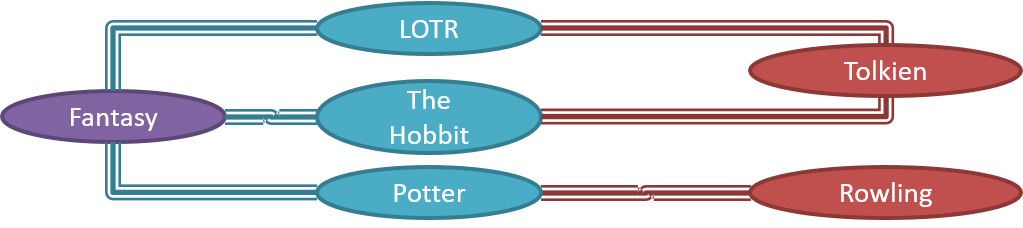Note that these object structures within the same software can change over time.

Given below is how the object structure in the previous example could have looked like at a different time.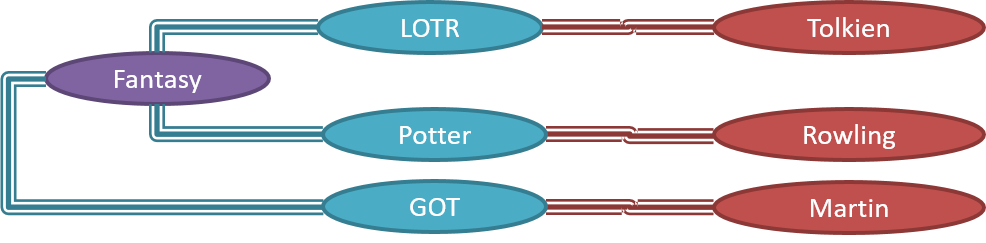However, object structures do not change at random; they change based on a set of rules, as was decided by the designer of that software. Those rules that object structures need to follow can be illustrated as a class structure  i.e. a structure that exists among the relevant classes.

Here is a class structure (drawn using an ad-hoc notation) that matches the object structures given in the previous two examples. For example, note how this class structure does not allow any connection between `Genre` objects and `Author` objects, a rule followed by the two object structures above.UML Object Diagrams are used to model object structures and UML Class Diagrams are used to model class structures of an OO solution.

Here is an object diagram for the above example: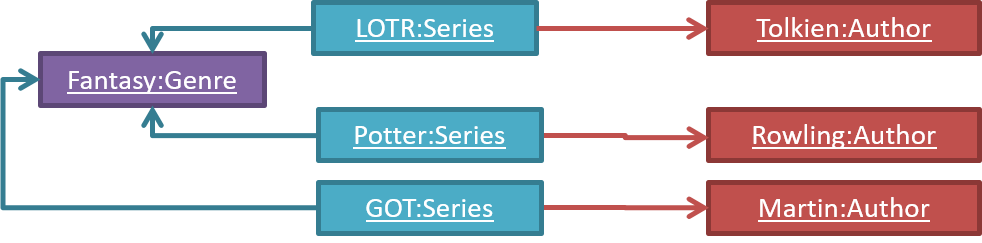And here is the class diagram for it: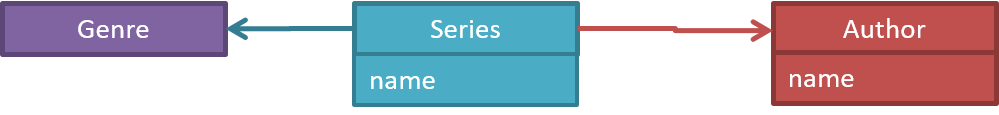Can use basic-level class diagrams

Classes form the basis of class diagrams.

Associations are the main connections among the classes in a class diagram.

The most basic class diagram is a bunch of classes with some solid lines among them to represent associations, such as this one.

An example class diagram showing associations between classes.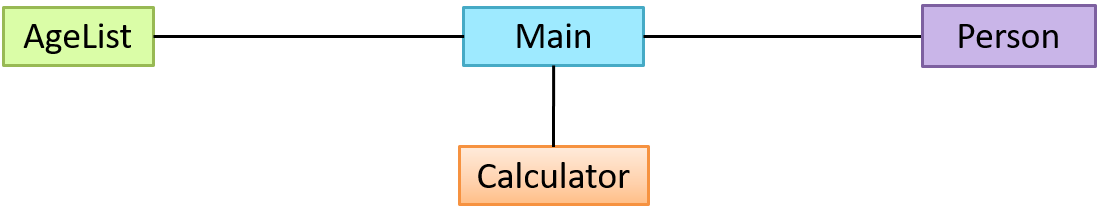Here is the same class diagram shown earlier but with some additional information included: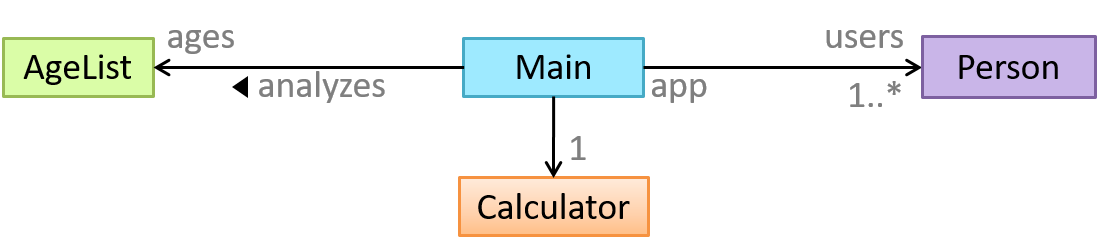Which association notatations are shown in this diagram?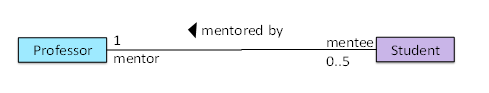• a. association labels
• b. association roles
• c. association multiplicity
• d. class names

(a) (b) (c) (d)

Explanation: '1’ is a multiplicity, ‘mentored by’ is a label, and ‘mentor’ is a role.

Explain the associations, navigabilities, and multiplicities in the class diagram below: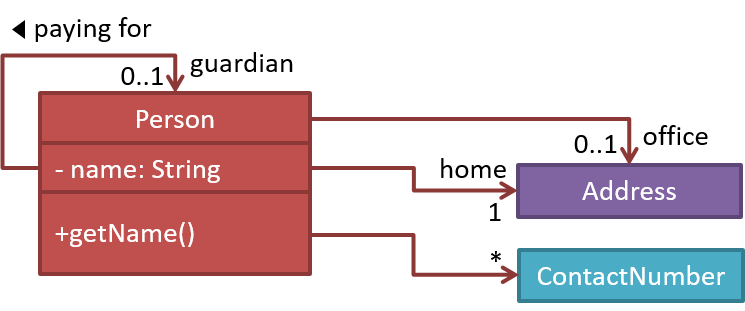Draw a class diagram for the code below. Show the attributes, methods, associations, navigabilities, and multiplicities in the class diagram below:

``````class Box {
private Item[] parts = new Item;
private Item spareItem;
private Lid lid; // lid of this box
private Box outerBox;

public void open(){
//...
}
}
``````
``````class Item {
public static int totalItems;
}
``````
``````class Lid {
Box box; // the box for which this is the lid
}
``````

Can use intermediate-level class diagrams

A class diagram can also show different types of associations: inheritance, compositions, aggregations, dependencies.

##### Modeling dependencies

A class diagram can also show different types of class-like entities:

##### Modeling interfaces

Which of these statements match the class diagram?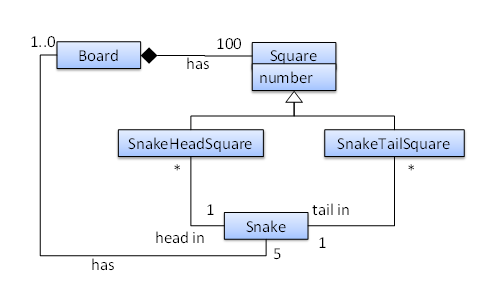• a. A `Snake` must belong to at least one `Board`.
• b. A `SnakeHeadSquare` can contain only one `Snake` head.
• c. A `Square` can contain a `Snake` head.
• d. A `Snake` head can be in more than one `SnakeHeadSquare`
• e. The `Board` has exactly 5 `Snake`s.

(a)(b)(c)(d)(e)

Explanation:

(a) does not match because a `Snake` may or may not belong to a `Board` (multiplicity is `0..1`)
(b) matches the diagram because the multiplicity given in `1`
(c) matches the diagram because `SnakeHeadSquare` is a `Square` (due to inheritance)
(d) matches the diagram because the multiplicity given is `*`
(e) matches the diagram because the multiplicity given in `5`

Explain the meaning of various class diagram notations in the following class diagram:Consider the code below:

``````public interface Billable {
void bill();
}
``````
``````public abstract class Item
implements Billable {
public abstract void print();
}
``````
``````public class StockItem extends Item {
private Review review;
private String name;

public StockItem(
String name, Rating rating){

this.name = name;
this.review = new Review(rating);
}

@Override
public void print() {
//...
}

@Override
public void bill() {
//...
}
}
``````
``````public enum Rating {
GOOD, OK, POOR
}
``````
``````public class Review {
private final Rating rating;

public Review(Rating rating) {
this.rating = rating;
}
}
``````
``````import java.util.List;

public class Inventory {
private List<Item> items;

public int getItemCount(){
return items.size();
}

public void generateBill(Billable b){
// ...
}

}
}
``````

(a) Draw a class diagram to represent the code
(b) Draw an object diagram to represent the situation where the inventory has one item with a name `spanner` and a review of `POOR` rating.

Can use basic object diagrams

Object diagrams can be used to complement class diagrams. For example, you can use object diagrams to model different object structures that can result from a design represented by a given class diagram.

This question is based on the following question from another topic:

Draw a class diagram for the code below. Show the attributes, methods, associations, navigabilities, and multiplicities in the class diagram below:

``````class Box {
private Item[] parts = new Item;
private Item spareItem;
private Lid lid; // lid of this box
private Box outerBox;

public void open(){
//...
}
}
``````
``````class Item {
public static int totalItems;
}
``````
``````class Lid {
Box box; // the box for which this is the lid
}
``````

Draw an object diagram to match the code. Include objects of all three classes in your object diagram.

Can explain object oriented domain models

The analysis process for identifying objects and object classes is recognized as one of the most difficult areas of object-oriented development. --Ian Sommerville, in the book Software Engineering

Class diagrams can also be used to model objects in the problem domain (i.e. to model how objects actually interact in the real world, before emulating them in the solution). Class diagrams are used to model the problem domain are called conceptual class diagrams or OO domain models (OODMs).

OO domain model of a snakes and ladders game is given below.

Description: Snakes and ladders game is played by two or more players using a board and a die. The board has 100 squares marked 1 to 100. Each player owns one piece. Players take turns to throw the die and advance their piece by the number of squares they earned from the die throw. The board has a number of snakes. If a player’s piece lands on a square with a snake head, the piece is automatically moved to the square containing the snake’s tail. Similarly, a piece can automatically move from a ladder foot to the ladder top. The player whose piece is the first to reach the 100th square wins.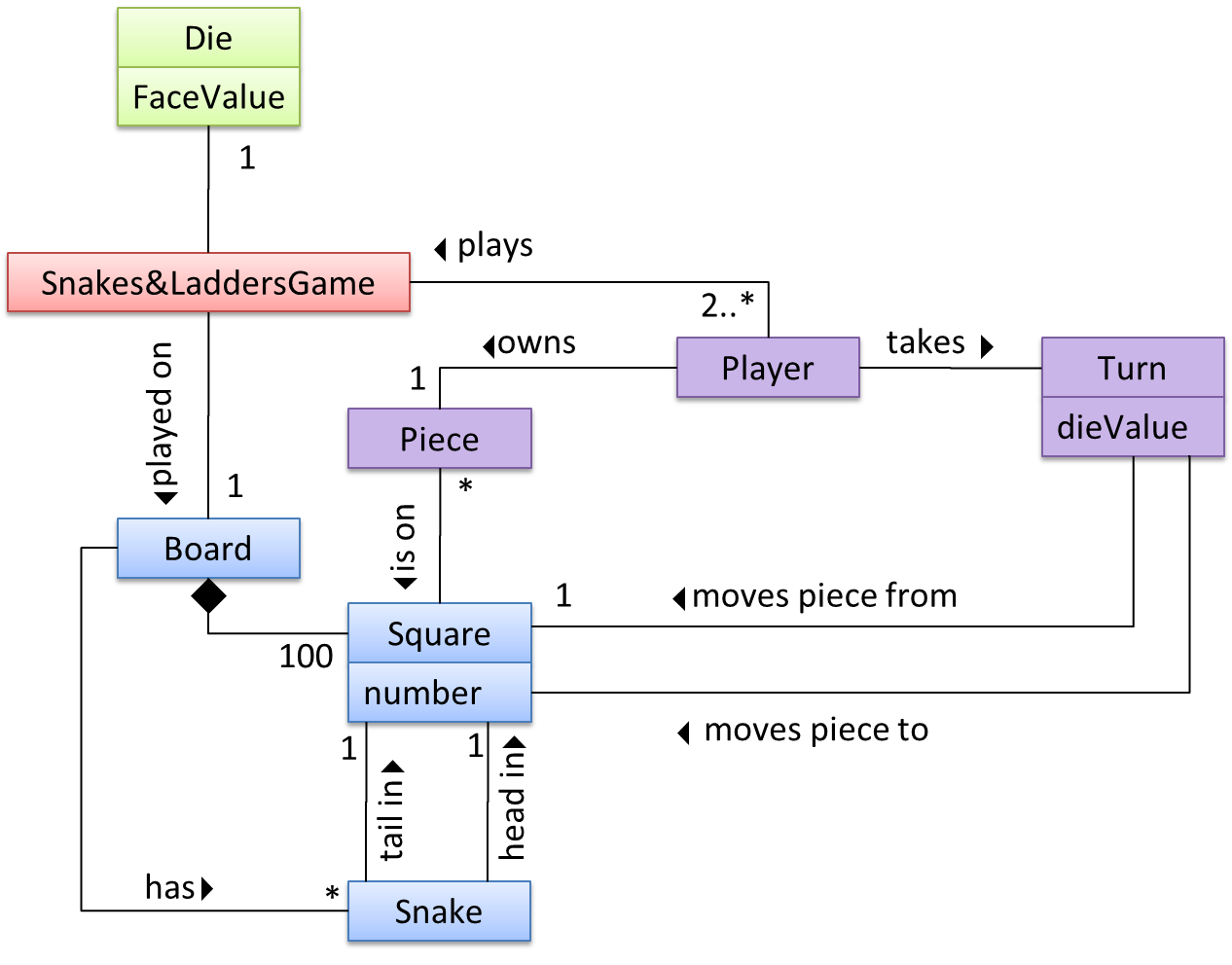The above OO domain model omits the ladder class for simplicity. It can be included in a similar fashion to the Snake class.

OODMs do not contain solution-specific classes (i.e. classes that are used in the solution domain but do not exist in the problem domain). For example, a class called DatabaseConnection could appear in a class diagram but not usually in an OO domain model because DatabaseConnection is something related to a software solution but not an entity in the problem domain.

OODMs represents the class structure of the problem domain and not their behavior, just like class diagrams. To show behavior, use other diagrams such as sequence diagrams.

OODM notation is similar to class diagram notation but typically omit methods and navigability.

This diagram is,

• a. A class diagram.
• b. An object diagram.
• c. An OO domain model, also known as a conceptual class diagram.
• d. Can be either a class diagram or an OO domain model.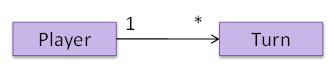(a)

Explanation: The diagram shows navigability which is not shown in an OO domain model. Hence, it has to be a class diagram.

What is the main difference between a class diagram and and an OO domain model?

(a)

Explanation: Both are UML diagrams, and use the class diagram notation. While it is true that often a class diagram may have more classes and more details, the main difference is that the OO domain model describes the problem domain while the class diagram describes the solution.

Can explain deployment diagrams

A deployment diagram shows a system's physical layout, revealing which pieces of software run on which pieces of hardware.

An example deployment diagram: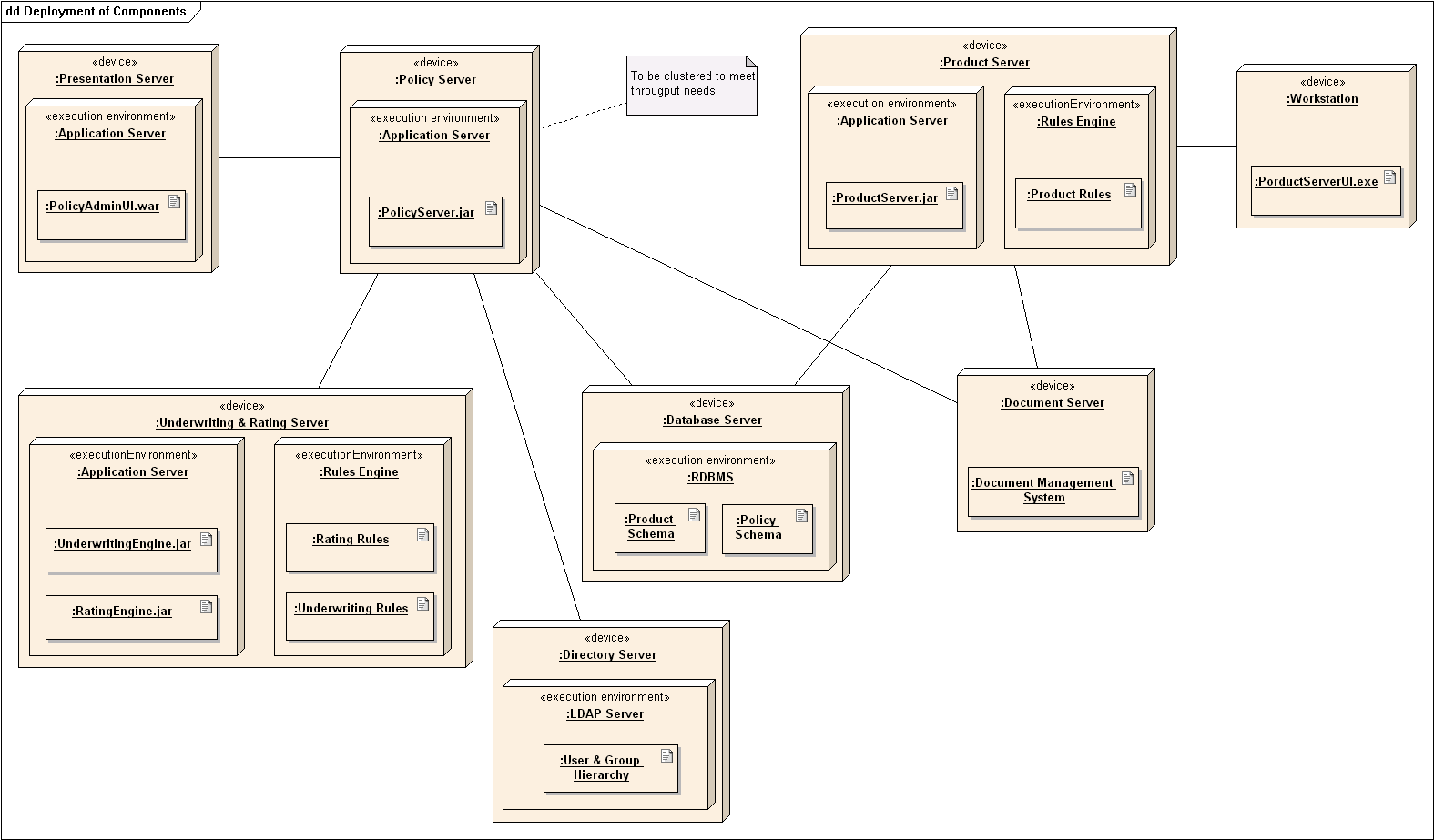source:https://commons.wikimedia.org

Can explain component diagrams

A component diagram is used to show how a system is divided into components and how they are connected to each other through interfaces.

An example component diagram:source:https://commons.wikimedia.org

Can explain package diagrams

A package diagram shows packages and their dependencies. A package is a grouping construct for grouping UML elements (classes, use cases, etc.).

Here is an example package diagram: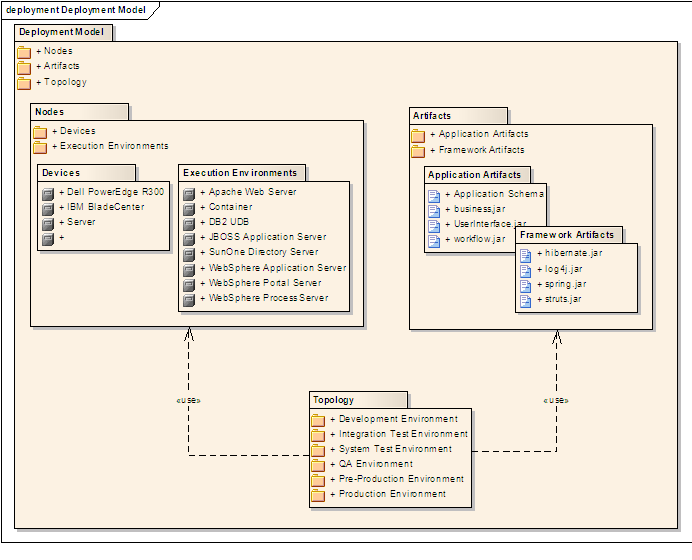source:https://commons.wikimedia.org

Can explain composite structure diagrams

A composite structure diagram hierarchically decomposes a class into its internal structure.

Here is an example composite structure diagram: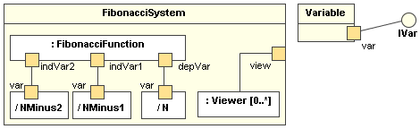source:https://commons.wikimedia.org

## Modeling Behaviors

Can use activity diagrams

Software projects often involve workflows. Workflows define the flow in which a process or a set of tasks is executed.

Some examples in which a certain workflow is relevant to software project:

A software that automates the work of an insurance company needs to take into account the workflow of processing an insurance claim.

The algorithm of a price of code represents the workflow (i.e. the execution flow) of the code.

Understanding such workflows is important for the success of the software project. UML  activity diagrams (AD) can model workflows.  Flow charts is another type of diagrams that can model workflows. Activity diagrams are the UML equivalent of flow charts.

Unified Modeling Language (UML) is a graphical notation to describe various aspects of a software system. UML is the brainchild of three software modeling specialists James Rumbaugh, Grady Booch and Ivar Jacobson (also known as the Three Amigos). Each of them has developed their own notation for modeling software systems before joining force to create a unified modeling language (hence, the term ‘Unified’ in UML). UML is currently the de facto modeling notation used in the software industry.

An example activity diagram [source:wikipeida]:The most basic activity diagram is simply a linear sequence of actions.

Some workflows have alternate paths where only one of the alternate paths is taken based on some condition.

In some workflows, multiple paths happen in parallel.

Which of these sequence of actions is not allowed by the given activity diagram?

• i. start a b c d end
• ii. start a b c d e f g c d end
• iii. start a b c d e g f c d end
• iv. start a b c d g c d end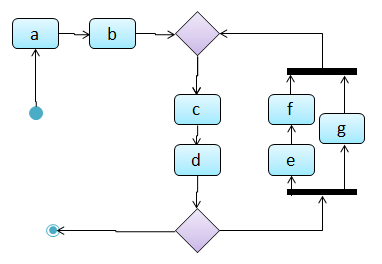(iv)

Explanation: `-e-f-` and `-g-` are parallel paths. Both paths should complete before the execution reaches `c` again.

Can draw basic sequence diagrams

Explain in your own words the interactions illustrated by this Sequence Diagram: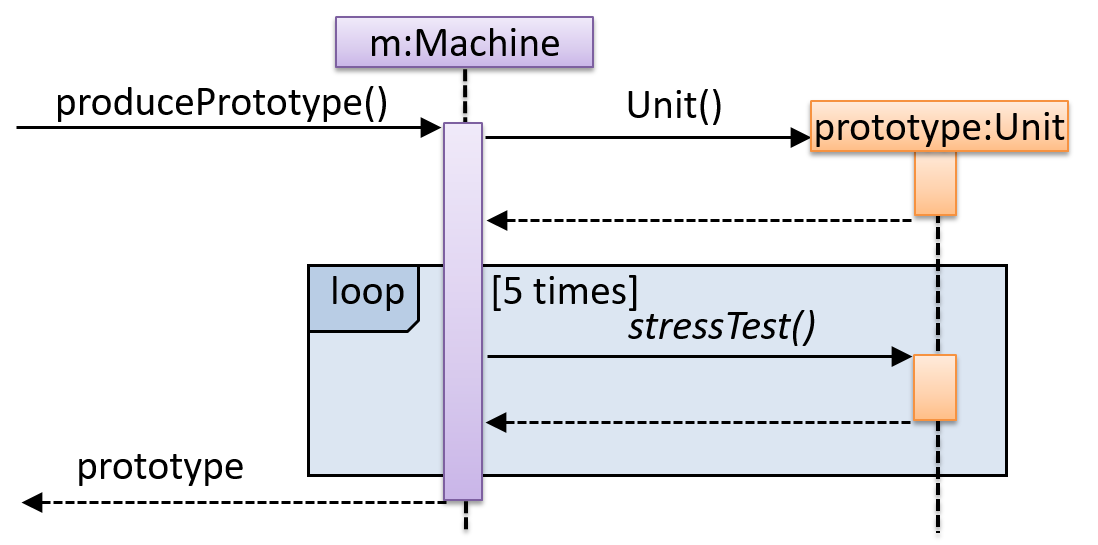Consider the code below:

``````class Person{
Tag tag;
String name;

Person(String personName, String tagName){
name = personName;
tag = new Tag(tagName);
}
}
``````

``````class Tag{
Tag(String value){
//...
}
}

class PersonList{
//...
}
}
``````

Draw a sequence diagram to illustrate the object interactions that happen in the code snippet below:

``````PersonList personList = new PersonList();
while (hasRoom){
Person p = new Person("Adam", "friend");
}
``````

Find notation mistakes in the sequence diagram below: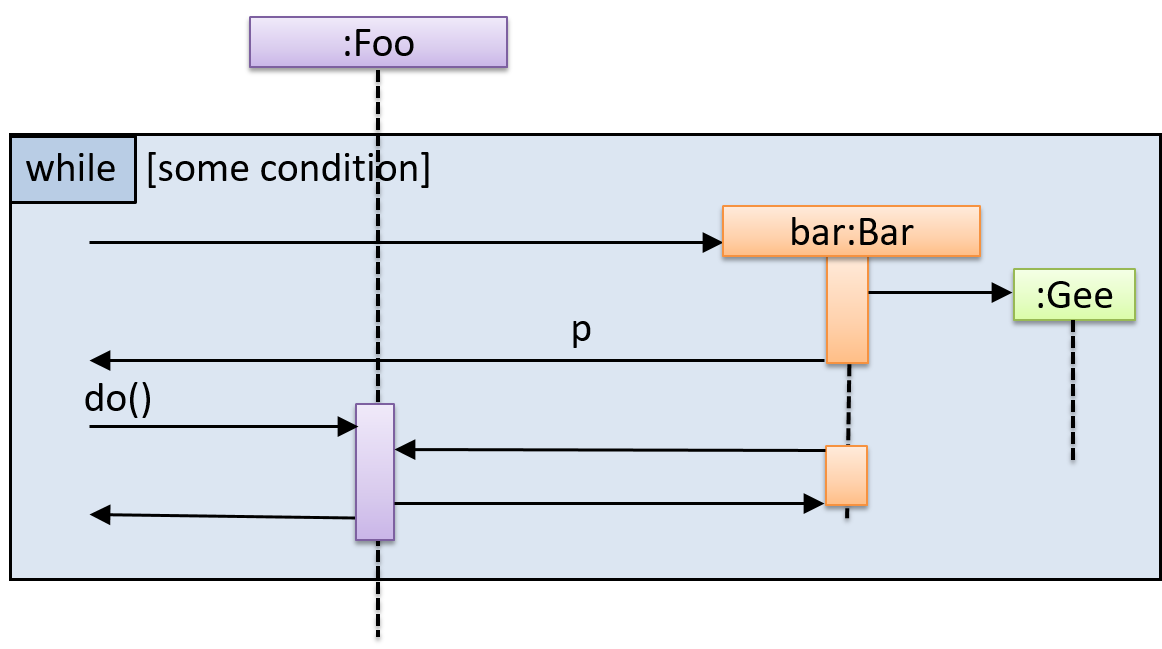Can draw intermediate-level sequence diagrams

What’s going on here?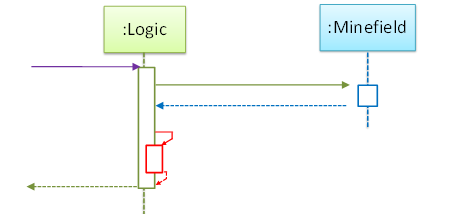• a. `Logic` object is executing a parallel thread.
• b. `Logic` object is executing a loop.
• c. `Logic` object is creating another `Logic` instance.
• d. One of `Logic` object’s methods is calling another of its methods.
• e. `Minefield` object is calling a method of `Logic`.

(d)

Explain the interactions depicted in this sequence diagram.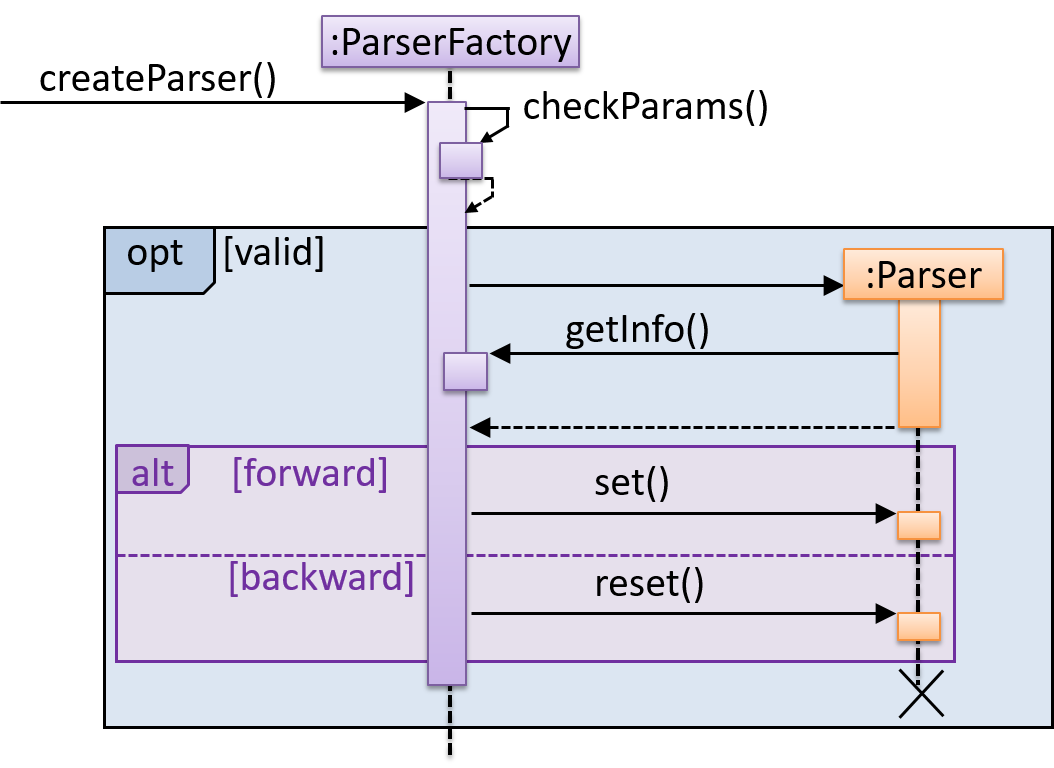First, the `createParser()` method of an existing `ParserFactory` object is called. Then, ...

Draw a sequence diagram to represent this code snippet.

``````if (isFirstPage) {
new Quote().print();
}
``````

The `Quote` class:

``````class Quote{

String q;

Quote(){
q = generate();
}

String generate(){
// ...
}

void print(){
System.out.println(q);
}

}
``````
• Show `new Quote().print();` as two method calls.
• As the created Quote object is not assigned to a variable, it can be considered as 'deleted' soon after its `print()` method is called.
Can explain use case diagrams

Use case diagrams model the mapping between features of a system and its user roles.

A simple use case diagram: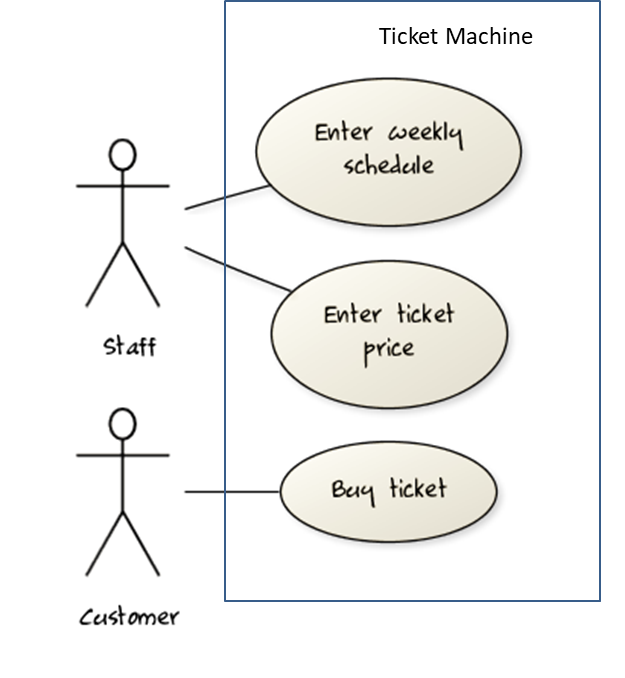Can explain timing diagrams

A timing diagram focus on timing constraints.

Here is an example timing diagram: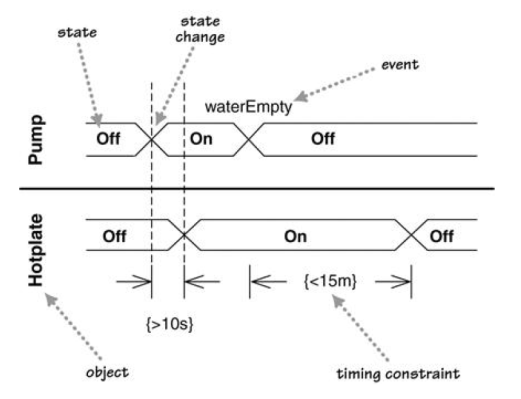Adapted from: UML Distilled by Martin Fowler

Can explain interaction overview diagrams

An Interaction overview diagrams is a combination of activity diagrams and sequence diagrams.

An example: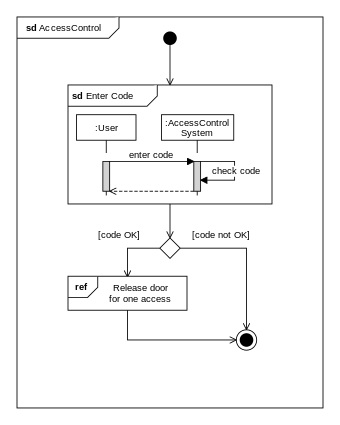source:https://commons.wikimedia.org

Can explain communication diagrams

A Communication diagrams are like sequence diagrams but emphasize the data links between the various participants in the interaction rather than the sequence of interactions.

An example: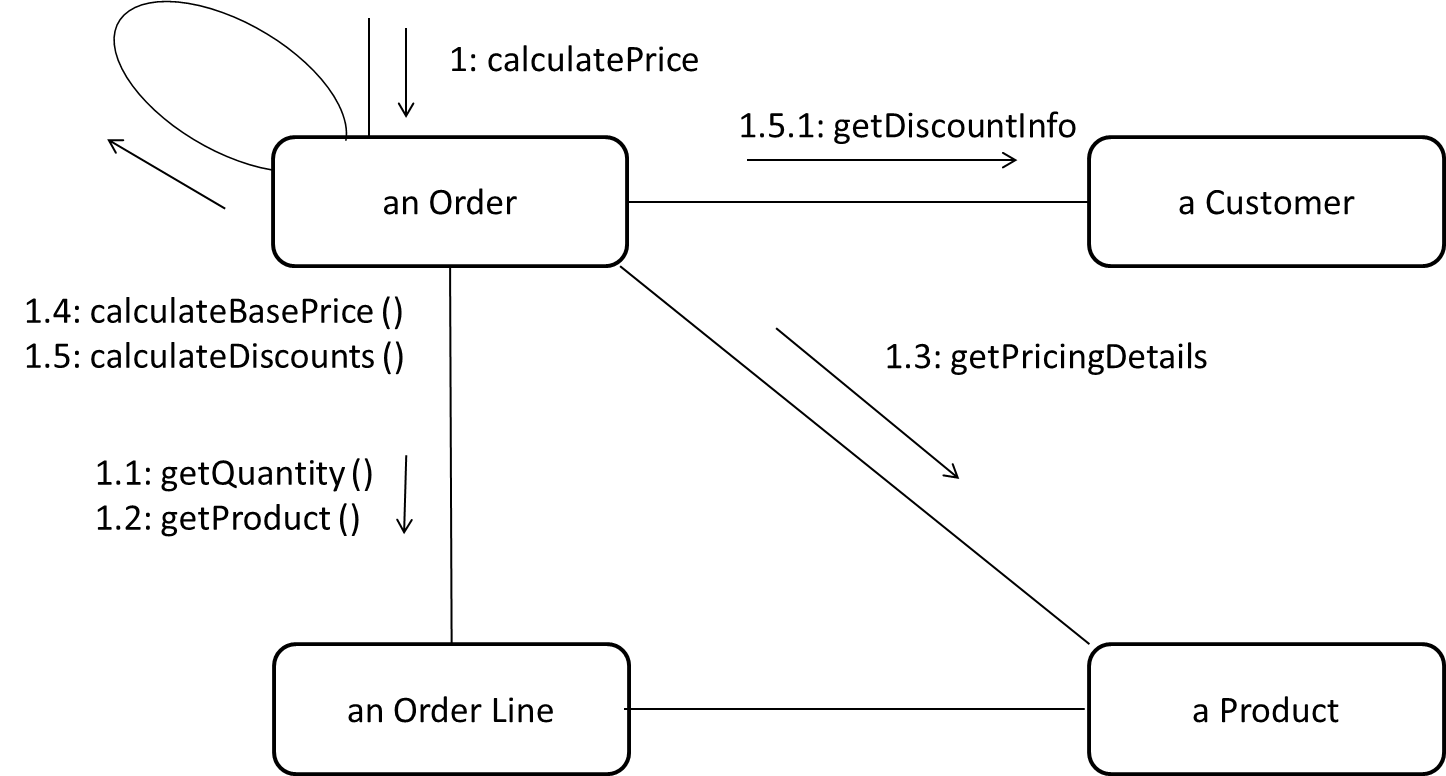Adapted from: UML Distilled by Martin Fowler

Can explain state machine diagrams

A State Machine Diagram models state-dependent behavior.

Consider how a CD player responds when the “eject CD” button is pushed:

• If the CD tray is already open, it does nothing.
• If the CD tray is already in the process of opening (opened half-way), it continues to open the CD tray.
• If the CD tray is closed and the CD is being played, it stops playing and opens the CD tray.
• If the CD tray is closed and CD is not being played, it simply opens the CD tray.
• If the CD tray is already in the process of closing (closed half-way), it waits until the CD tray is fully closed and opens it immediately afterwards.

What this means is that the CD player’s response to pushing the “eject CD” button depends on what it was doing at the time of the event. More generally, the CD player’s response to the event received depends on its internal state. Such a behavior is called a state-dependent behavior.

Often, state-dependent behavior displayed by an object in a system is simple enough that it needs no extra attention; such a behavior can be as simple as a conditional behavior like `if x>y, then x=x-y`. Occasionally, objects may exhibit state-dependent behavior that is complex enough such that it needs to be captured into a separate model. Such state-dependent behavior can be modelled using UML state machine diagrams (SMD for short, sometimes also called ‘state charts’, ‘state diagrams’ or ‘state machines’).

An SMD views the life-cycle of an object as consisting of a finite number of states where each state displays a unique behavior pattern. An SMD captures information such as the states an object can be in, during its lifetime, and how the object responds to various events while in each state and how the object transits from one state to another. In contrast to sequence diagrams that capture object behavior one scenario at a time, SMDs capture the object’s behavior over its full life cycle.

An SMD for the Minesweeper game.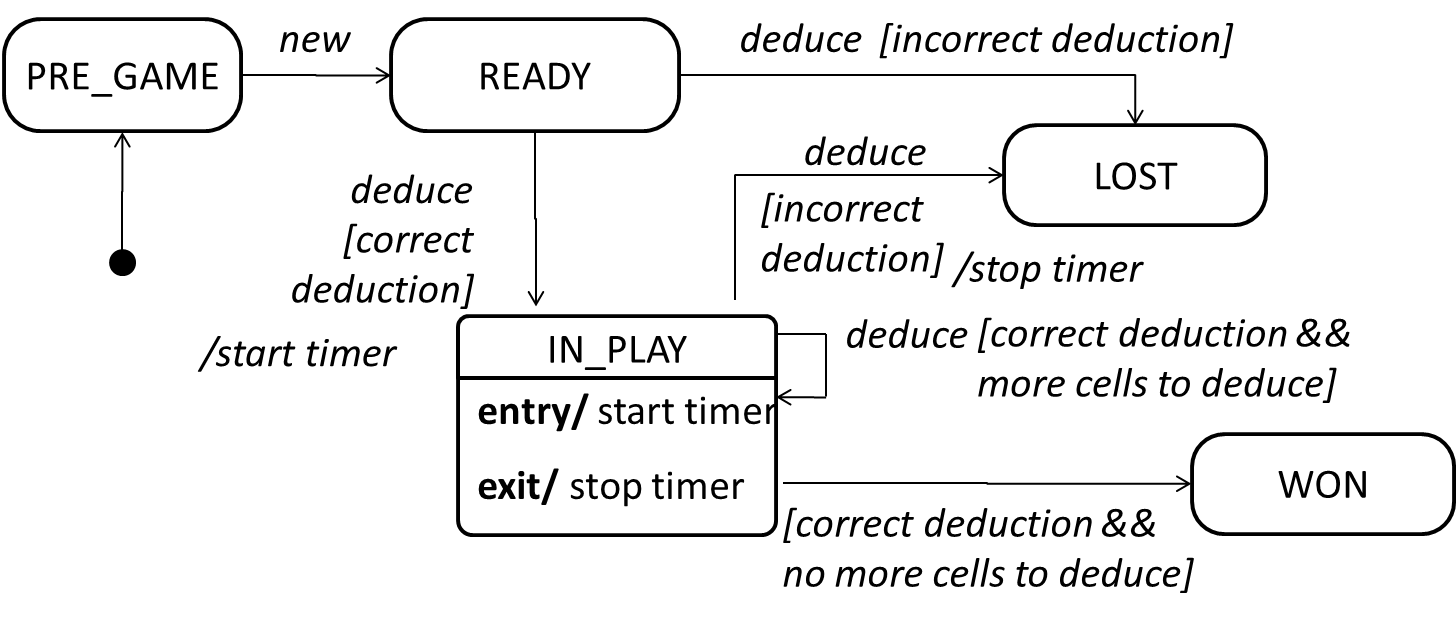## Modeling a Solution

Can explain how modelling can be used before implementation

You can use models to analyze and design a software before you start coding.

Suppose You are planning to implement a simple minesweeper game that has a text based UI and a GUI. Given below is a possible OOP design for the game.Before jumping into coding, you may want to find out things such as,

• Is this class structure is able to produce the behavior we want?
• What API should each class have?
• Do we need more classes?

To answer those questions, you can analyze the how the objects of these classes will interact with each other to produce the behavior you want.

Can use simple class diagrams and sequence diagrams to model an OO solution

As mentioned in [ Design → Modeling → Modeling a Solutions → Introduction], this is the Minesweeper design you have come up with so far. Our objective is to analyze, evaluate, and refine that design.

Design → Modeling → Modeling a Solution →

#### Introduction

You can use models to analyze and design a software before you start coding.

Suppose You are planning to implement a simple minesweeper game that has a text based UI and a GUI. Given below is a possible OOP design for the game.Before jumping into coding, you may want to find out things such as,

• Is this class structure is able to produce the behavior we want?
• What API should each class have?
• Do we need more classes?

To answer those questions, you can analyze the how the objects of these classes will interact with each other to produce the behavior you want.Let us start by modelling a sample interaction between the person playing the game and the TextUi object.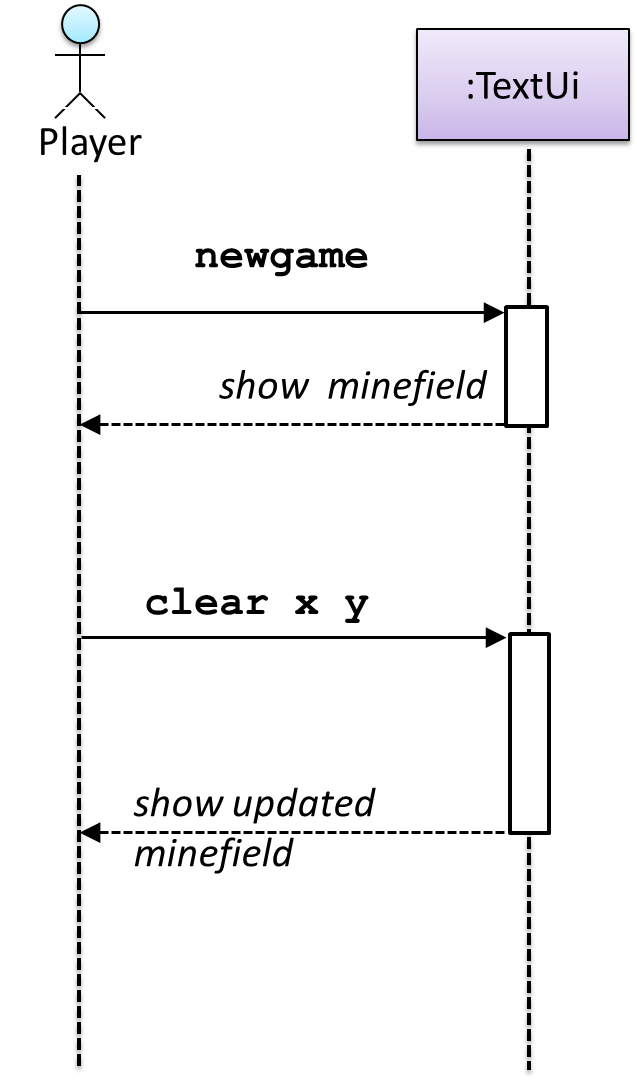`newgame` and `clear x y` represent commands typed by the `Player` on the `TextUi`.

How does the `TextUi` object carry out the requests it has received from player? It would need to interact with other objects of the system. Because the `Logic` class is the one that controls the game logic, the `TextUi` needs to collaborate with `Logic` to fulfill the `newgame` request. Let us extend the model to capture that interaction.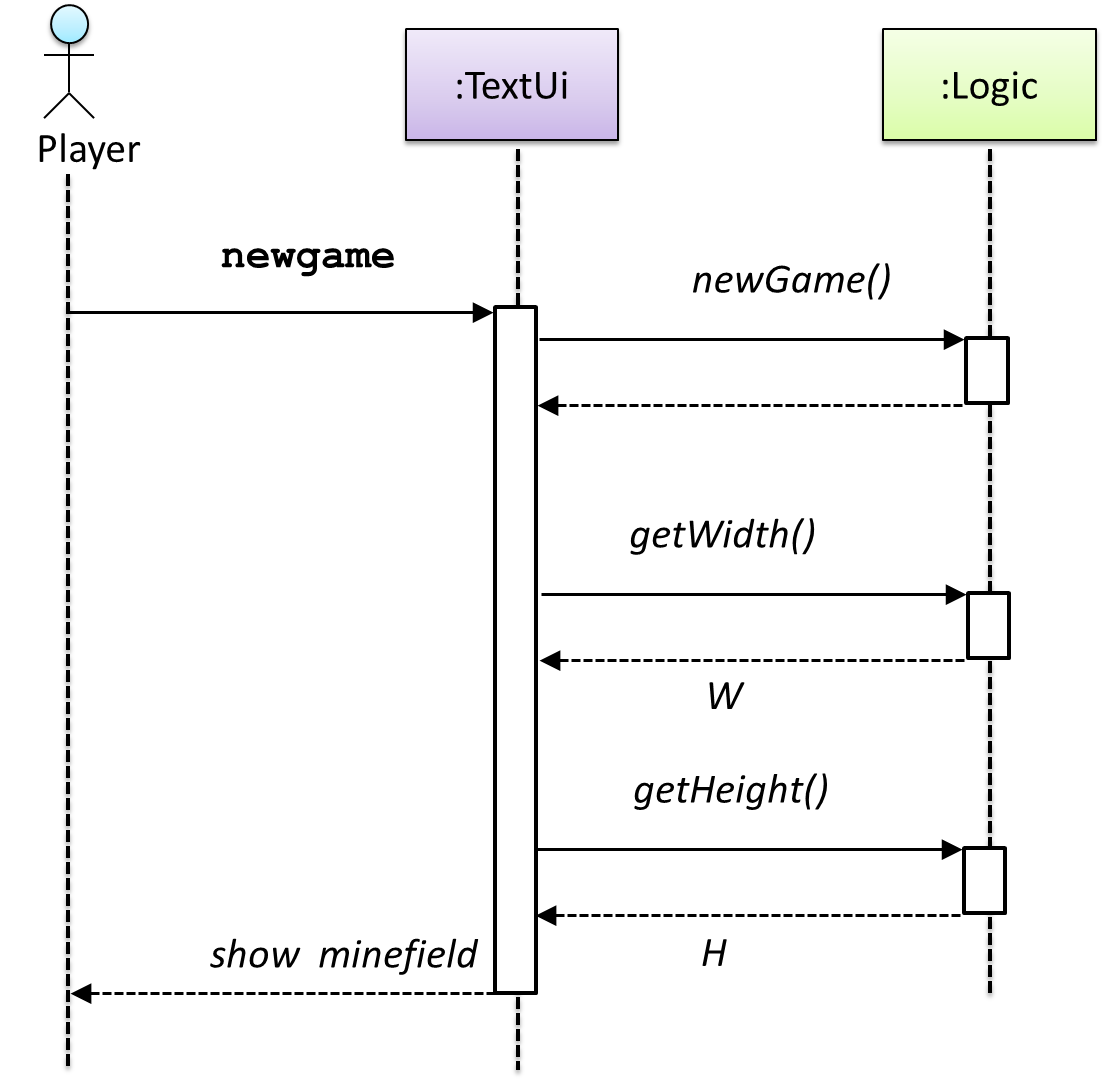W = Width of the minefield; H = Height of the minefield

The above diagram assumes that `W` and `H` are the only information `TextUi` requires to display the minefield to the `Player`. Note that there could be other ways of doing this.
The `Logic` methods we conceptualized in our modelling so far are: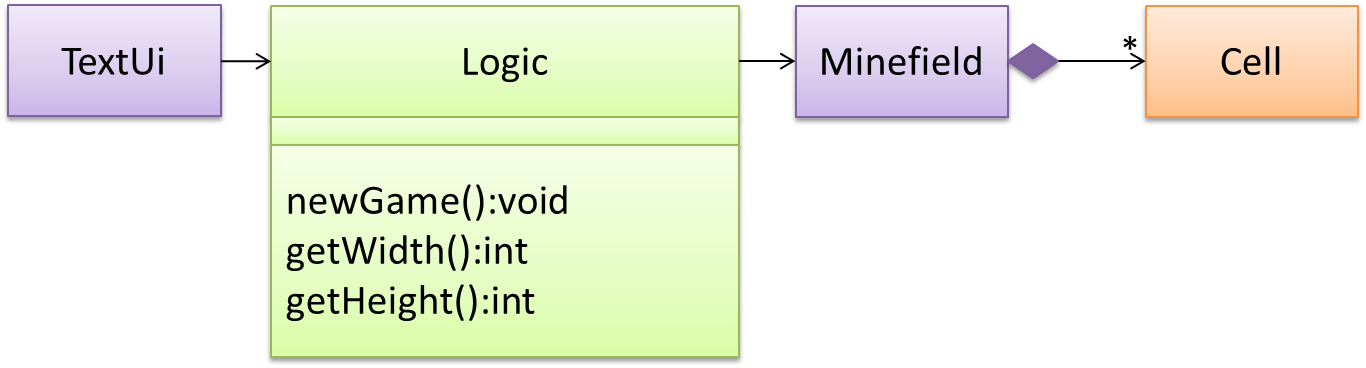Now, let us look at what other objects and interactions are needed to support the `newGame()` operation. It is likely that a new `Minefield` object is created when the `newGame()` method is called.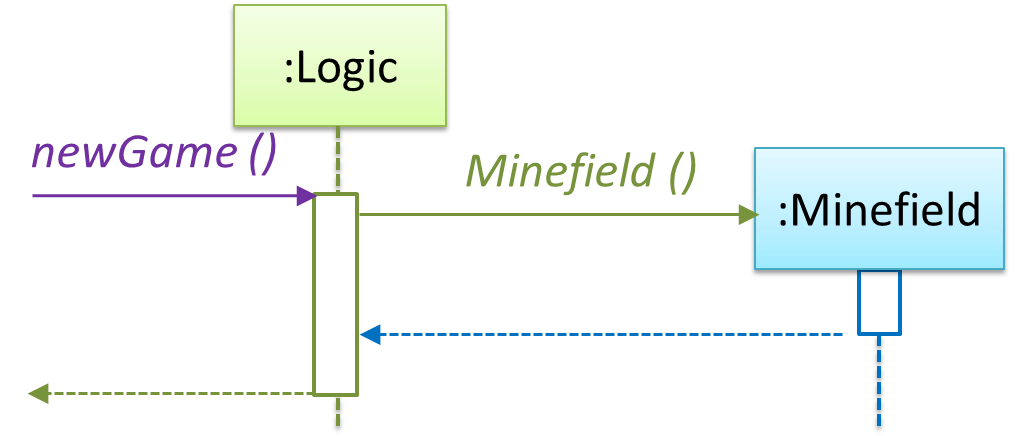Note that the behavior of the `Minefield` constructor has been abstracted away. It can be designed at a later stage.

Given below are the interactions between the player and the Text UI for the whole game.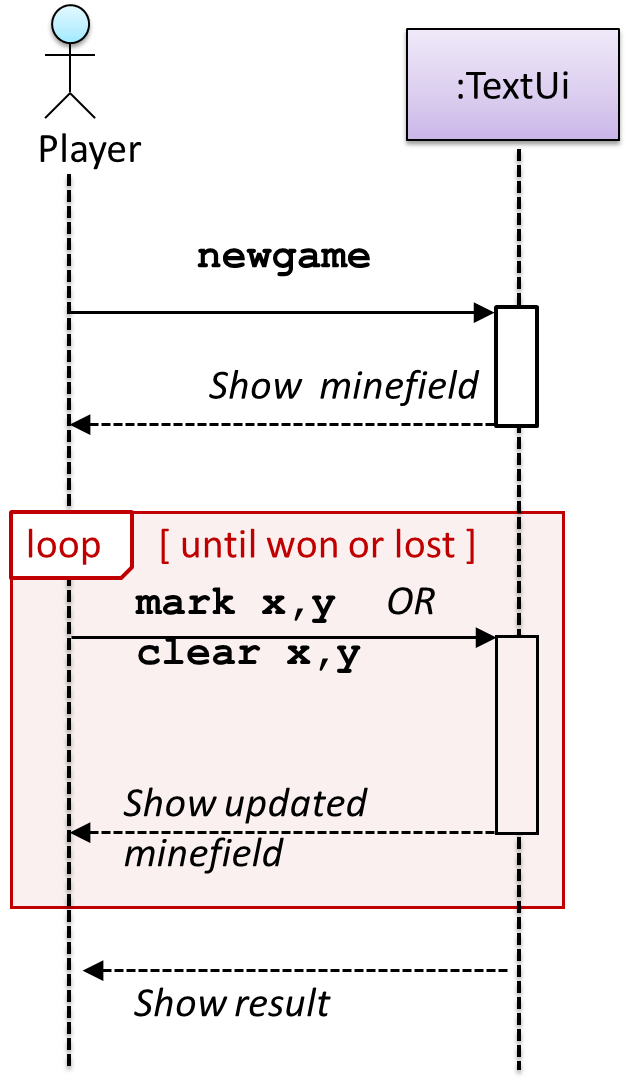💡 Note that a similar technique can be used when discovering/defining the architecture-level APIs.

Defining the architecture-level APIs for a small Tic-Tac-Toe game:

Can use intermediate class diagram and sequence diagram concepts to model an OO design

Continuing with the example in [ Design → Modeling → Modeling a Solution → Basic], next let us model how the TextUi interacts with the Logic to support the mark or clear operations until the game is won or lost.

Design → Modeling → Modeling a Solution →

#### Basic

As mentioned in [ Design → Modeling → Modeling a Solutions → Introduction], this is the Minesweeper design you have come up with so far. Our objective is to analyze, evaluate, and refine that design.

Design → Modeling → Modeling a Solution →

#### Introduction

You can use models to analyze and design a software before you start coding.

Suppose You are planning to implement a simple minesweeper game that has a text based UI and a GUI. Given below is a possible OOP design for the game.Before jumping into coding, you may want to find out things such as,

• Is this class structure is able to produce the behavior we want?
• What API should each class have?
• Do we need more classes?

To answer those questions, you can analyze the how the objects of these classes will interact with each other to produce the behavior you want.Let us start by modelling a sample interaction between the person playing the game and the TextUi object.`newgame` and `clear x y` represent commands typed by the `Player` on the `TextUi`.

How does the `TextUi` object carry out the requests it has received from player? It would need to interact with other objects of the system. Because the `Logic` class is the one that controls the game logic, the `TextUi` needs to collaborate with `Logic` to fulfill the `newgame` request. Let us extend the model to capture that interaction.W = Width of the minefield; H = Height of the minefield

The above diagram assumes that `W` and `H` are the only information `TextUi` requires to display the minefield to the `Player`. Note that there could be other ways of doing this.
The `Logic` methods we conceptualized in our modelling so far are:Now, let us look at what other objects and interactions are needed to support the `newGame()` operation. It is likely that a new `Minefield` object is created when the `newGame()` method is called.Note that the behavior of the `Minefield` constructor has been abstracted away. It can be designed at a later stage.

Given below are the interactions between the player and the Text UI for the whole game.💡 Note that a similar technique can be used when discovering/defining the architecture-level APIs.

Defining the architecture-level APIs for a small Tic-Tac-Toe game: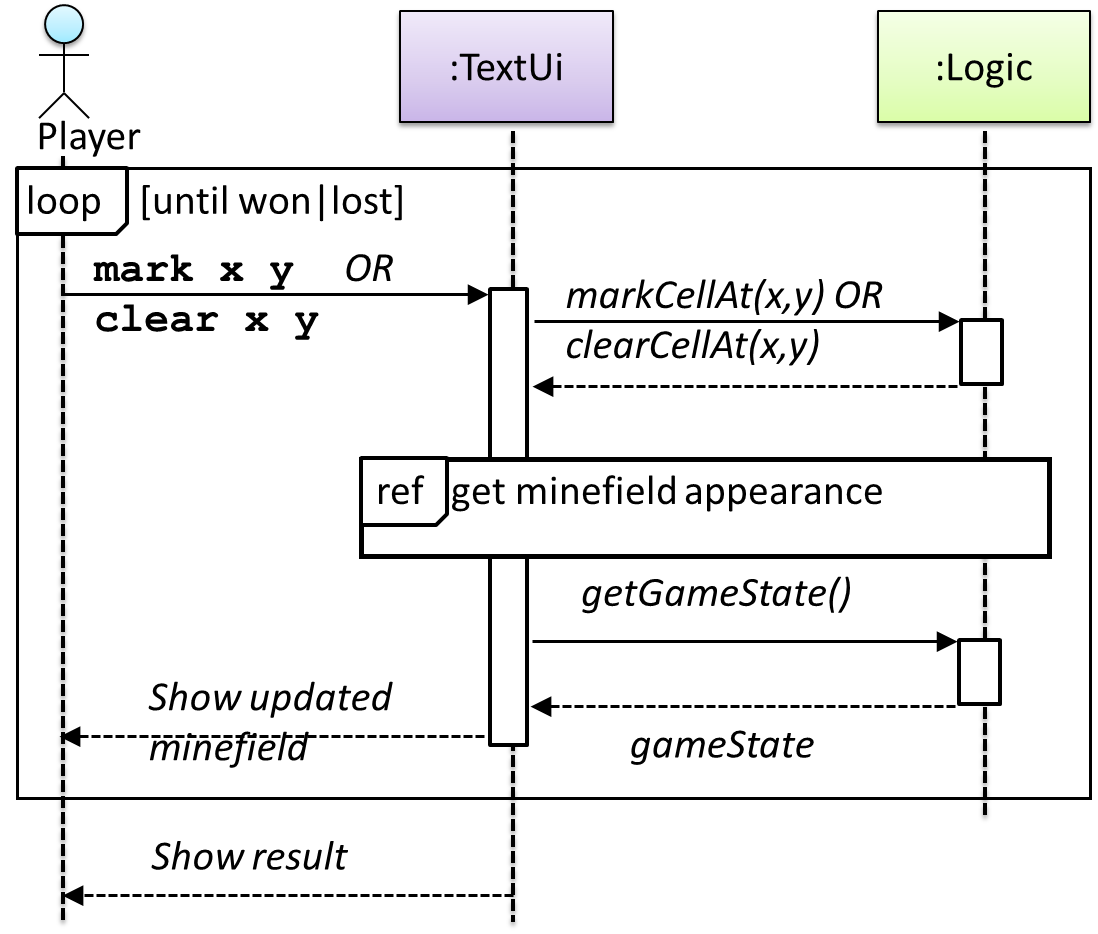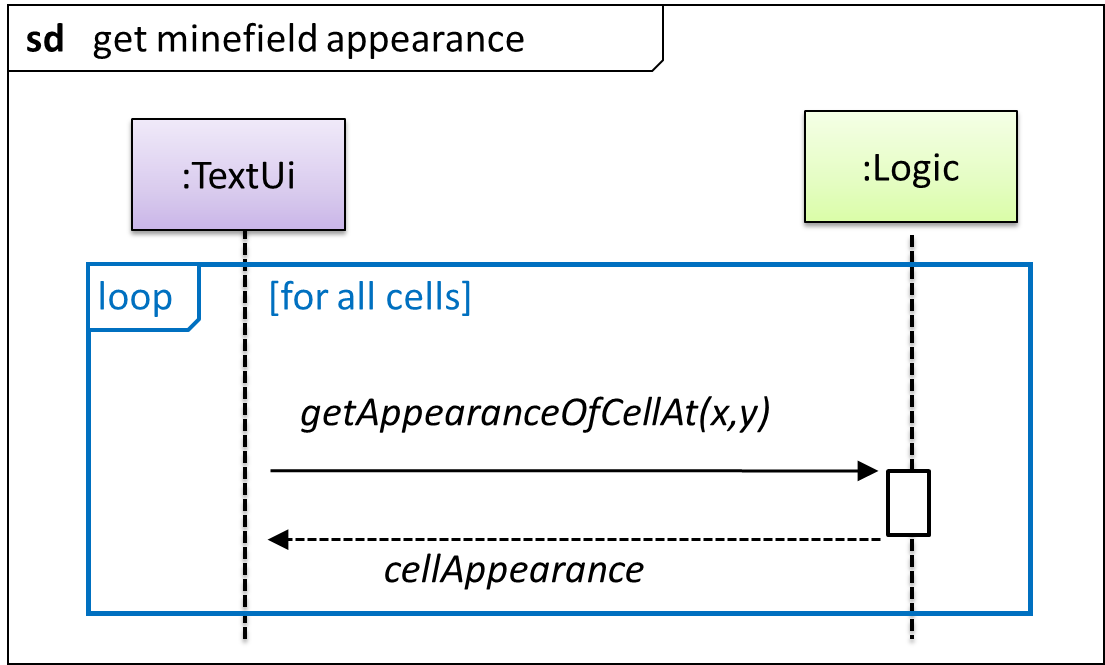This interaction adds the following methods to the `Logic` class

• `clearCellAt(int x, int y)`
• `markCellAt(int x, int y)`
• `getGameState() :GAME_STATE (GAME_STATE: READY, IN_PLAY, WON, LOST, …)`

And it adds the following operation to Logic API:

• `getAppearanceOfCellAt(int,int):CELL_APPEARANCE (CELL_APPEARANCE: HIDDEN, ZERO, ONE, TWO, THREE, …, MARKED, INCORRECTLY_MARKED, INCORRECTLY_CLEARED)`

In the above design, `TextUi` does not access `Cell` objects directly. Instead, it gets values of type `CELL_APPEARANCE` from `Logic` to be displayed as a minefield to the player. Alternatively, each cell or the entire Minefield can be passed directly to `TextUi`.

Here is the updated class diagram: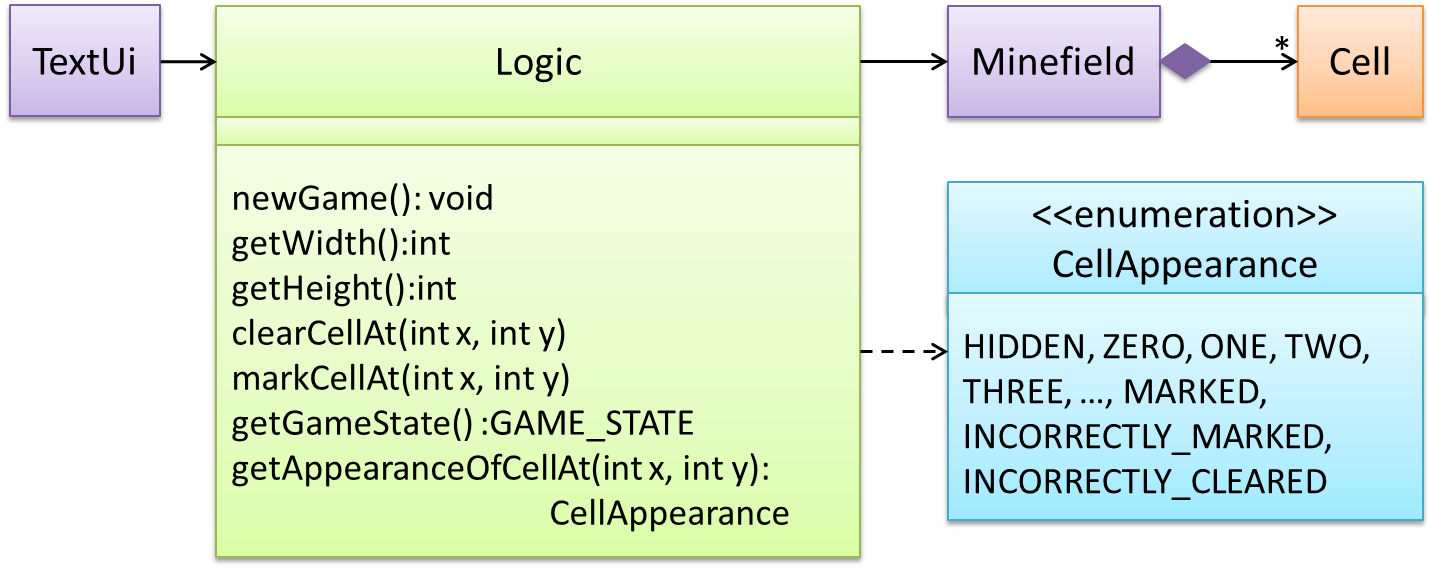The above is for the case when Actor `Player` interacts with the system using a text UI. Additional operations (if any) required for the GUI can be discovered similarly. Suppose `Logic` supports a `reset()` operation. We can model it like this: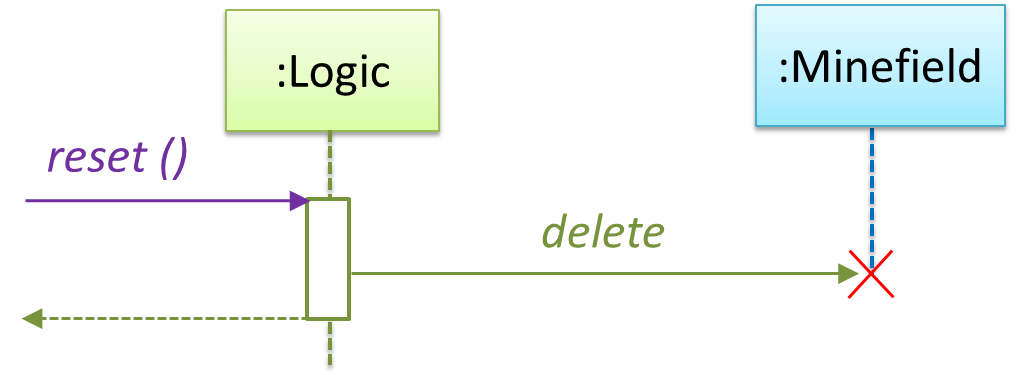Our current model assumes that the `Minefield` object has enough information (i.e. H, W, and mine locations) to create itself.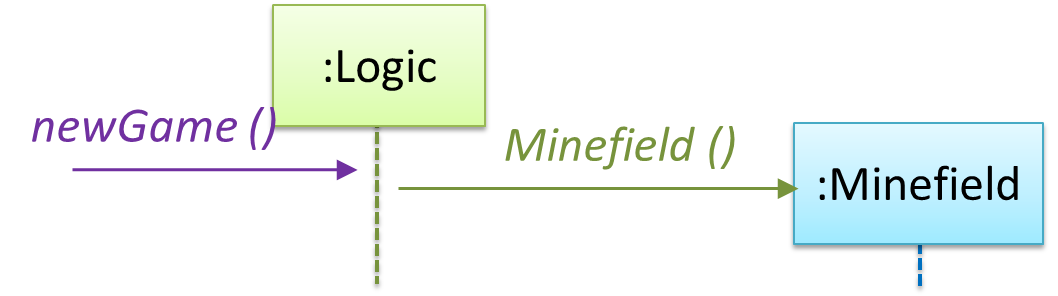An alternative is to have a `ConfigGenerator` object that generates a string containing the minefield information as shown below.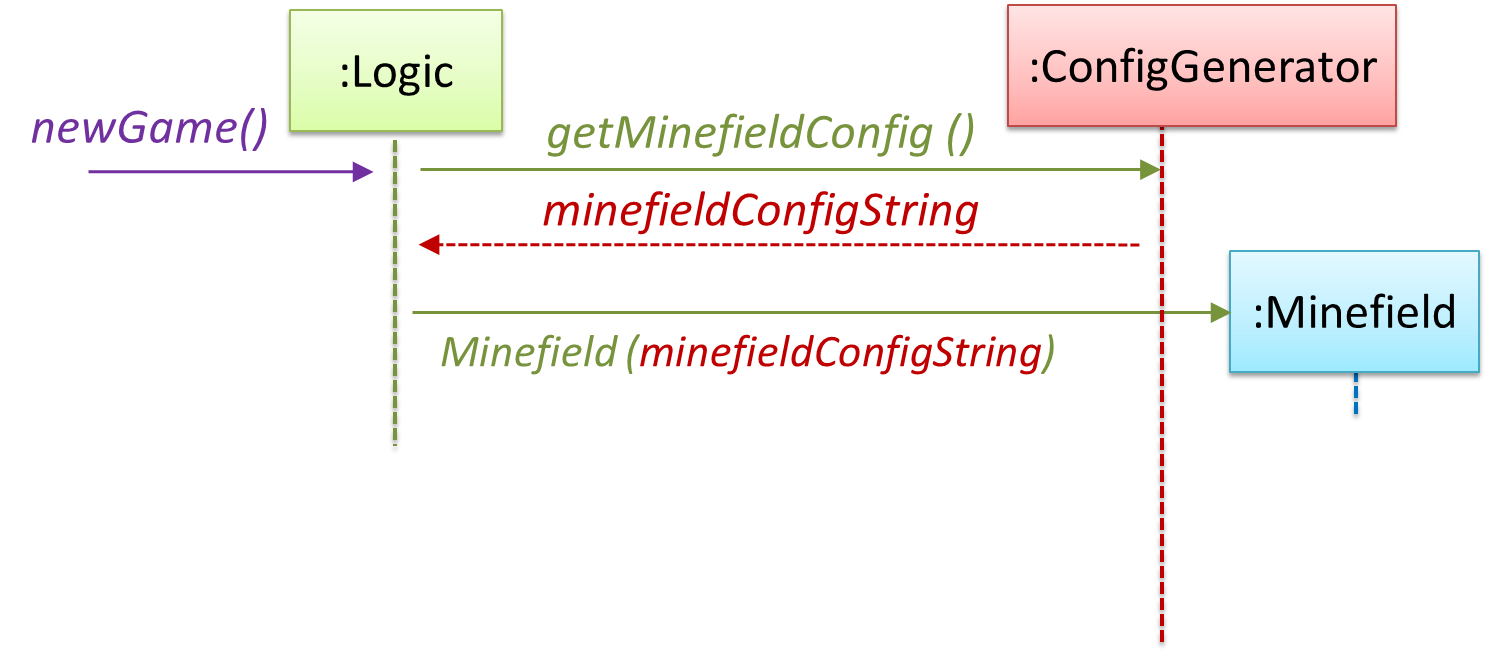In addition, `getWidth()`, `getHeight()`, `markCellAt(x,y)` and `clearCellAt(x,y)` can be handled like this.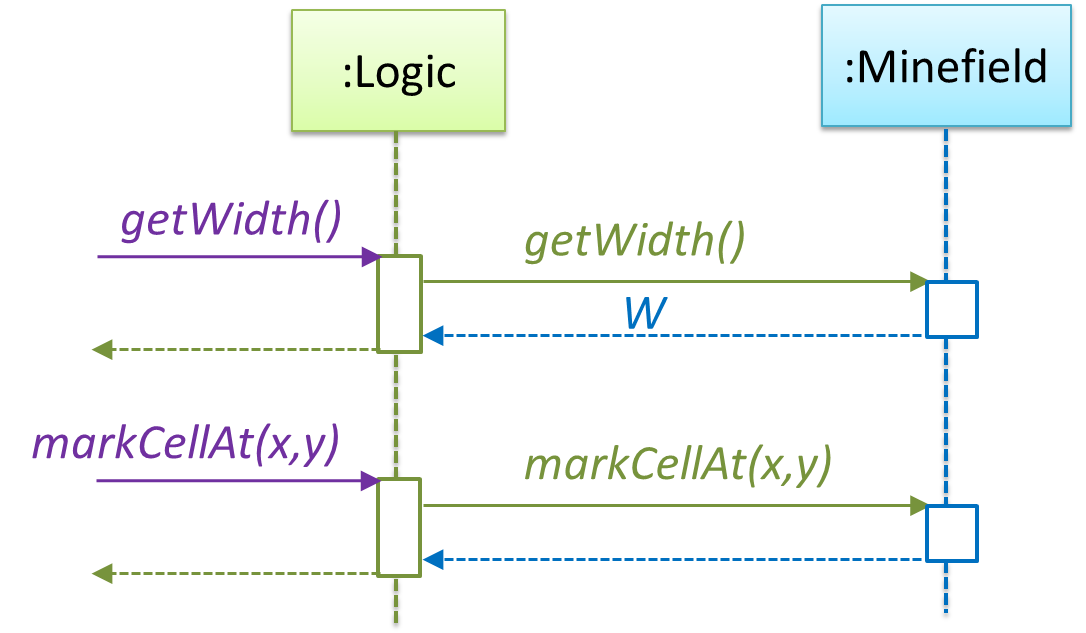The updated class diagram: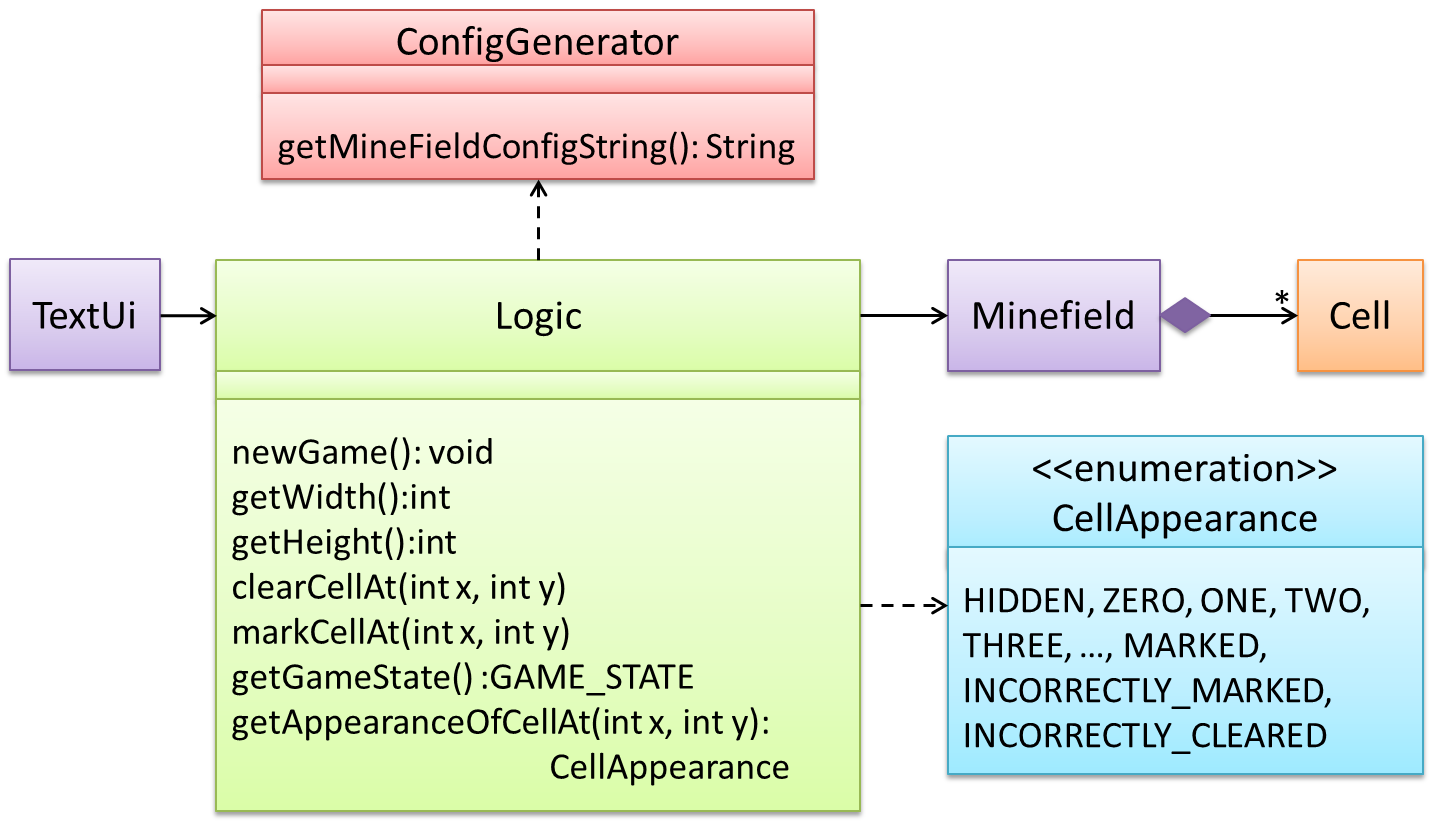How is `getGameState()` operation supported? Given below are two ways (there could be other ways):

1. `Minefield` class knows the state of the game at any time. `Logic` class retrieves it from the `Minefield` class as and when required.
2. `Logic` class maintains the state of the game at all times.

Here’s the SD for option 1.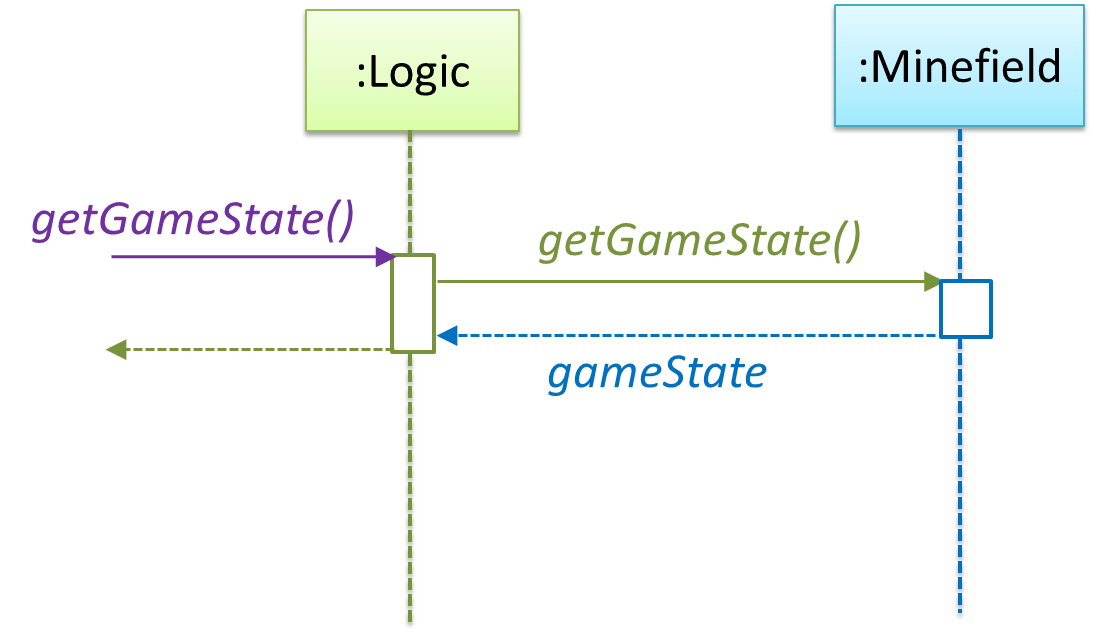Here’s the SD for option 2. Here, assume that the game state is updated after every mark/clear action.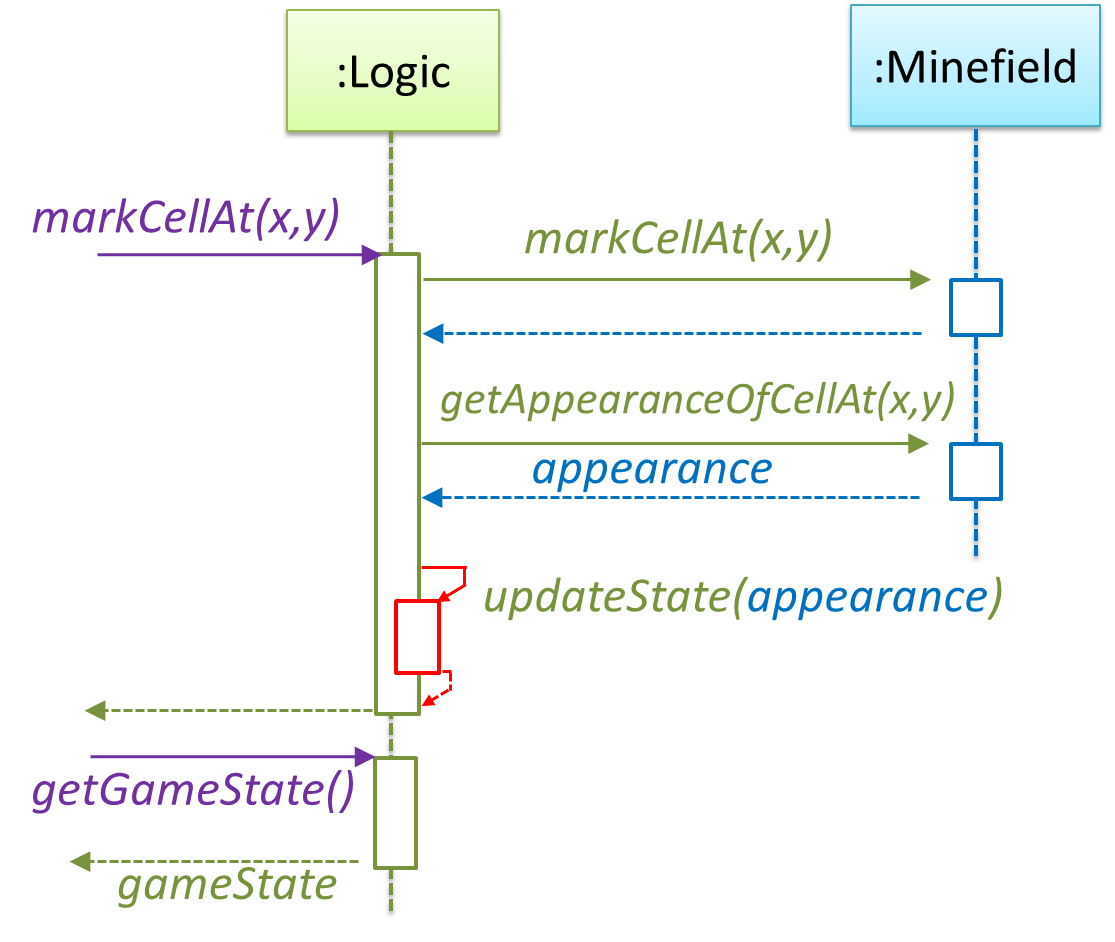It is now time to explore what happens inside the `Minefield` constructor? One way is to design it as follows.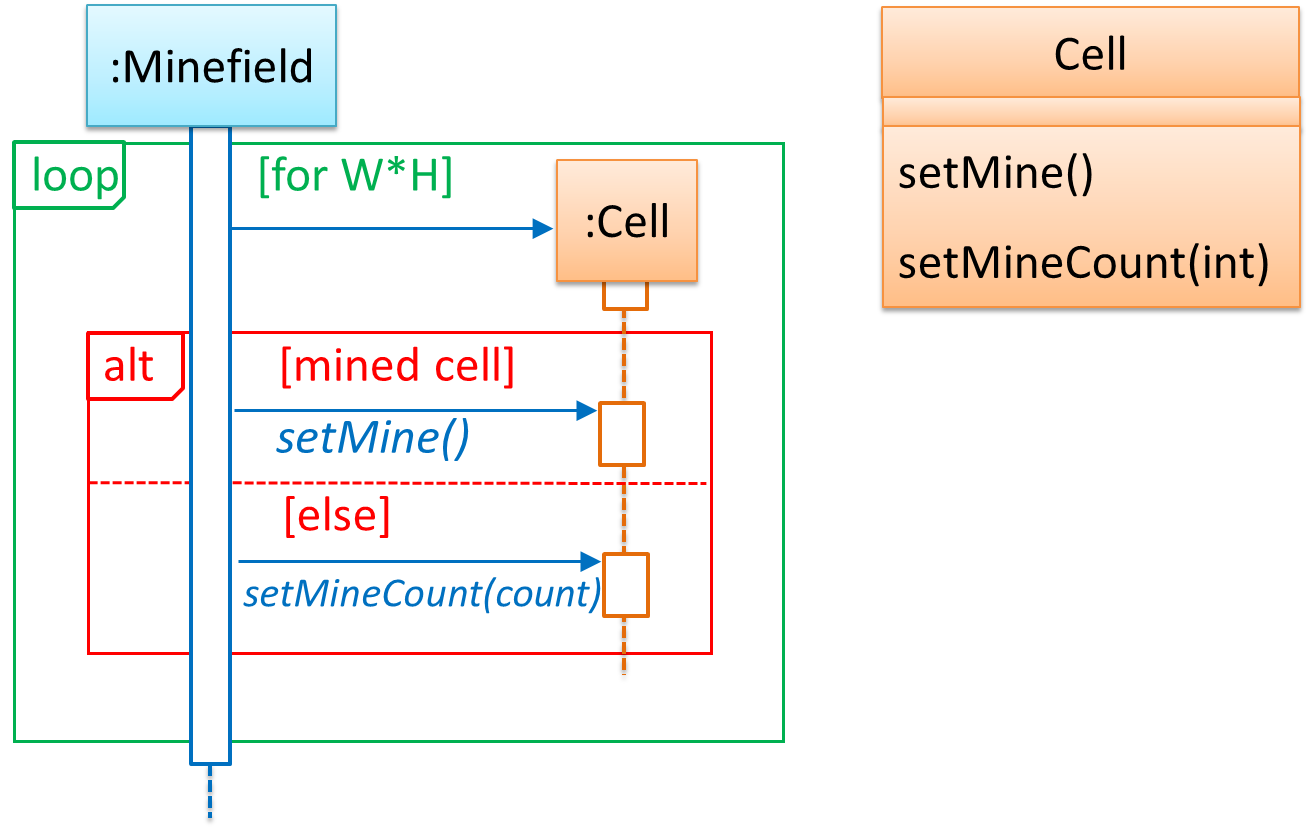Now let us assume that `Minesweeper` supports a ‘timing’ feature.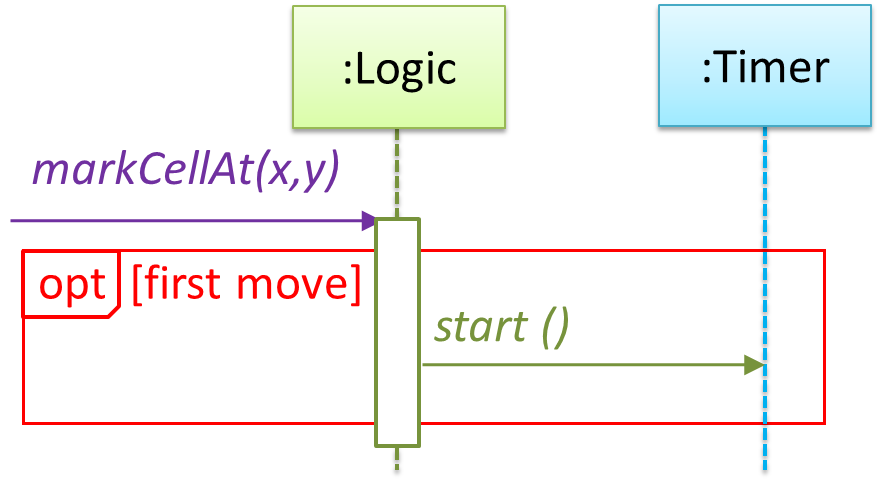Updated class diagram: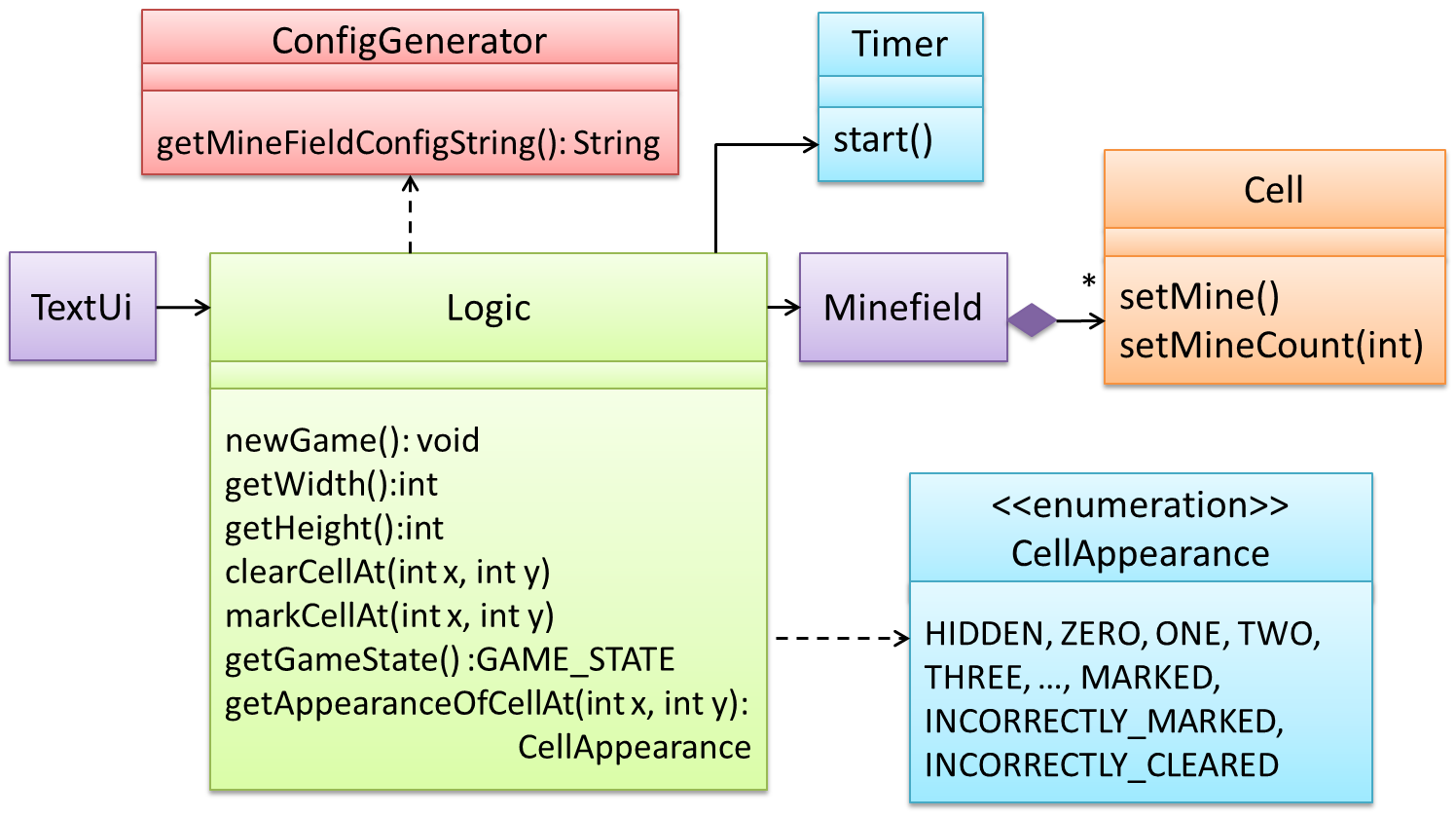💡 When designing components, it is not necessary to draw elaborate UML diagrams capturing all details of the design. They can be done as rough sketches. For example, draw sequence diagrams only when you are not sure which operations are required by each class, or when you want to verify that your class structure can indeed support the required operations.